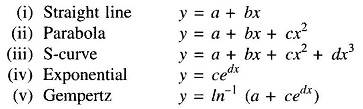## Types of Forecasting Methods:

Types of Forecasting Methods may be divided into three broad classes. Techniques may be based on extrapolation or on correlation or on a combination of both. Techniques may be further classified as either deterministic, probabilistic or stochastic.

### Extrapolation Techniques:

Extrapolation techniques involve fitting trend curves to basic historical data adjusted to reflect the growth trend itself. With a trend curve the forecast is obtained by evaluating the trend curve function at the desired future point. Although a very simple procedure, it produces reasonable results in some instances. Such a types of forecasting methods is called a deterministic extrapolation since random errors in the data or in analytical model are not accounted for.

Standard analytical functions used in trend curve fitting areThe most common curve-fitting technique for fitting coefficients and exponents (a-d) of a function in a given forecast is the method of least squares. If the uncertainty of extrapolated results is to be quantified using statistical entities such as mean and variance, the basic technique becomes probabilistic extrapolation. With regression analysis the best estimate of the model describing the trend can be obtained and used to forecast the trend.

### Correlation Techniques:

Correlation techniques of forecasting relate system loads to various demographic and economic factors. This approach is advantageous in forcing the forecaster to understand clearly the interrelationship between load growth patterns and other measurable factors. The disadvantage is the need to forecast demographic and economic factors, which can be more difficult than forecasting system load. Typically, such factors as population, employment, building permits, business, weather data and the like are used in correlation techniques.

No one forecasting method is effective in all situations. Forecasting Methodology must be used as tools to aid the planner; good judgement and experience can never be completely replaced.

Scroll to Top